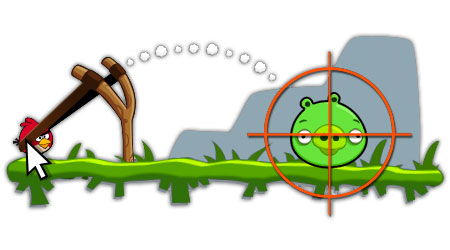# Hitting a Bird with Projectile...A stone is projected from a point on the ground in such a direction as to hit a bird on the top of a telegraph post of height $H$ and then attain maximum height of $2H$ above the ground. If at the instant of projection, the bird were to fly away horizontally with uniform speed, Find the ratio between the horizontal velocity of bird and the stone, if the stone still hits the bird while descending.
The answer is of the form $\frac{a}{b\sqrt{c} + d}$. Then enter the answer as $a + b + c + d$, where $a, b, c, d$ are integers.

×

Problem Loading...

Note Loading...

Set Loading...